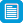# Search results

## Refine by topic

Main topic Specific topic
Related topic
Listed under:  Mathematics  >  Number (Mathematics)  >  Commutativity### TIMES Module 3: Number and Algebra: multiplication and division - teacher guide

This is a 23-page guide for teachers. This module contains a description of suitable models for multiplication, a discussion of the type of problem phrased in words that requires multiplication for its solution, and mental and written strategies for multiplication. The use of the commutative, associative and distributive ...### TIMES Module 9: Number and Algebra: multiplication of whole numbers - teacher guide

This is a 23-page guide for teachers. This module contains a description of suitable models for multiplication, a discussion of the types of problems that require multiplication for their solution, and mental and written strategies for multiplication. The use of the commutative, associative and distributive laws is described. ...### TIMES Module 2: Number and Algebra: addition and subtraction - teacher guide

This is a 29-page guide for teachers. The module introduces addition and subtraction of whole numbers.### TIMES Module 10: Number and Algebra: division of whole numbers - teacher guide

This is a 26-page guide for teachers. This module contains a description of suitable models for division, a discussion of the types of problems that require division for their solution, and mental and written strategies for division.### Mental computation

This is a website designed for both teachers and students that discusses methods of mental computation. In particular, applying the associative, commutative and distributive laws to aid mental and written computation is discussed. These are important ideas for the introduction of algebra. There are pages for both teachers ...### TIMES Module 7: Number and Algebra: addition of whole numbers - teacher guide

This is a 16-page guide for teachers. This module introduces addition of whole numbers.### The laws of arithmetic and their use in algebra

This is a website designed for both teachers and students that refers to algebraic notation, the laws of arithmetic and the use of these laws in algebra from the Australian Curriculum for year 7 students. It contains material on algebraic notation, the commutative and associative laws, the use of brackets and the orders ...### The number partner: go figure

This tutorial is suitable for use with a screen reader. It explains strategies for breaking up numbers into pairs of smaller numbers, eg 15 = 11 + 4. Work through examples of whole number pairs and sample questions. Apply these principles to solve additions or subtractions.### The difference bar: go figure

This tutorial is suitable for use with a screen reader. It explains how to split up numbers in your head when finding the difference between two numbers such as 26 and 73. Work through sample questions and instructions explaining how to use linear partitioning techniques. Find the difference between pairs of numbers. Split ...### The divider: with or without remainders

Solve divisions such as 147/7 or 157/6 (some have remainders). Use a partitioning tool to help solve randomly generated divisions. Learn strategies to do complex arithmetic in your head. Split a division into parts that are easy to work with, use times tables, then solve the original calculation.### The difference bar: generate easy subtractions

Learn how to split up numbers in your head. Use a linear partitioning tool to help find the difference between pairs of two-digit numbers such as 25 and 34. In these examples, the difference is always less than ten. Split the numbers into parts that are easy to work with, work out each part and then solve the original calculation.### The array: go figure

This tutorial is suitable for use with a screen reader. It explains strategies for solving simple multiplications in your head such as 6x4. Work through sample questions and instructions explaining how to break up numbers into their factors. Solve multiplications by using arrays to break them up into rows and columns, then ...### The multiplier: go figure

This tutorial is suitable for use with a screen reader. It explains strategies for solving complex multiplications in your head such as 22x38. Work through sample questions and instructions explaining how to use partitioning techniques. Solve multiplications by breaking them up into parts that are easy to work with, use ...